• Pandas模块是python中很重要的一个存在，它可以将同一索引下的数据进行合并，帮助大家更好的进行数据分析！最初是用于金融行业数据分析，后来得以在各个行业中广泛运行。软件介绍Pandas是python的一个数据分析包，...

Pandas模块是python中很重要的一个存在，它可以将同一索引下的数据进行合并，帮助大家更好的进行数据分析！最初是用于金融行业数据分析，后来得以在各个行业中广泛运行。

软件介绍

Pandas是python的一个数据分析包，最初由AQR Capital Management于2008年4月开发，并于2009年底开源出来，目前由专注于Python数据包开发的PyData开发team继续开发和维护，属于PyData项目的一部分。Pandas最初被作为金融数据分析工具而开发出来，因此，pandas为时间序列分析提供了很好的支持。 Pandas的名称来自于面板数据(panel data)和python数据分析(data analysis)。panel data是经济学中关于多维数据集的一个术语，在Pandas中也提供了panel的数据类型。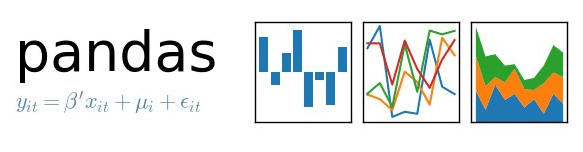Python中pandas模块快速入门

我这里简单介绍一下Python中，pandas模块定义的两种常用的数据结构，Series和DaraFrame。Series和Python中的Dict类似，但是是结构化的，而DataFrame和数据库中的表类似。

1.pandas基本数据结构-pandas.Series&pandas.DataFrame

第二种定义DataFrame的方法不能手动设置index，只能自动生成序列。

date_range('20180201',periods=8)定义时间序列，可以用freq来设置频率：s=pd.date_range('20180101',periods=8,freq='h')

支持的频率有s、min、h、d、w、m、y，默认为d

2.pandas查看数据

3.pandas常规操作

更新日志

包括许多API更改、新特性、增强和性能改进，以及大量bug修复。

亮点包括:

删除Python 2支持

使用重新标记的Groupby聚合

更好的多指标报告

更好的截断repr的系列和数据aframe

展开全文• numpy库有两个作用：​1、区别于list列表，提供了数组操作、数组运算、以及统计分布和简单的数学模型​2、计算速度快，甚至要优于python内置的简单运算，使得其成为pandas、sklearn等模块的依赖包。高级的框架如...

一、numpy模块

1.1 numpy 简介

numpy是python的一种开源的数值计算扩展库，这种库可用来存储和处理大型numpy数组。

numpy库有两个作用：

​1、区别于list列表，提供了数组操作、数组运算、以及统计分布和简单的数学模型

​2、计算速度快，甚至要优于python内置的简单运算，使得其成为pandas、sklearn等模块的依赖包。高级的框架如TensorFlow、PyTorch等，其数组操作也和numpy非常相似。

1.2 创建numpy数组

numpy数组即numpy的ndarray对象，创建numpy数组就是把一个列表传入np.array()方法

import numpy as np

# 创建一维的ndarray对象

arr =np.array([1,2,3])

print(arr,type(arr)) # [1 2 3]

# 创建二维的ndarray对象

print(np.array([[1,2,3],[4,5,6]]))

--------------------------------------------------------------------------------

[[1 2 3]

[4 5 6]]

1.3 numpy数组的常用属性

属性

解释

T

数组的转置(对高维数组而言)

dtype

数组元素的数据类型

size

数组元素的个数

ndim

数组的维数

shape

数组的维度大小(以元组形式)

astype

类型转换

arr = np.array([[1,2,3],[4,5,6]])

print(arr.T) # 行与列互换

--------------------------------------------------------------------------------

[[1 4]

[2 5]

[3 6]]

1.4 切片

arr = np .array([[1,2,3],[4,5,6]])

print(arr[:]) # 取出数组所有元素

print(arr[:,:]) # 取出数组所有元素

print(arr[0,:]) # 取出第0行到第一行的数组

print(arr[0:1,:]) # 取出第0行到第一行的数组，顾头不顾尾

print(arr[0:1,0:1]) # 取出第0行到第一行，第0列到第一列的数组，顾头不顾尾

print(arr[0, 0],type(arr[0, 0])) #取出第0行到第一行，第0列到第一列的数，输出数组类型

print(arr[0, [0,2]]) #取出第0行第0个元素和第2个元素 [1 3]

print(arr[0, 0] + 1) #取出第0行第0列的元素加1 2

1.5 取值

arr = np.array([[1, 2, 3], [4, 5, 6]])

arr[0, :] = 0 #将第0行的元素全部变为0

print(arr)

--------------------------------------------

[[0 0 0]

[4 5 6]]

arr[1, 1] = 1 #将第一行第一列的数字改为1

print(arr)

--------------------------------------------------------------------------------

[[0 0 0]

[4 1 6]]

arr[arr < 3] = 3 # 布尔取值 将小于3的数字全部变为3

print(arr)

--------------------------------------------------------------------------------

[[3 3 3]

[4 3 6]]

1.6 合并

arr1 = np.array([[1, 2, 3], [4, 5, 6]]) # 可变数据类型

arr2 = np.array([[7, 8, 9], [10, 11, 12]]) # 可变数据类型

-------------------------------------------------------

[[1 2 3]

[4 5 6]]

[[ 7 8 9]

[10 11 12]]

print(np.hstack((arr1,arr2))) # 行合并

------------------------------------------------------------

[[ 1 2 3 7 8 9]

[ 4 5 6 10 11 12]]

print(np.vstack((arr1,arr2))) # 列合并

------------------------------------------------

[[ 1 2 3]

[ 4 5 6]

[ 7 8 9]

[10 11 12]]

print(np.concatenate((arr1, arr2))) # 默认列合并

print(np.concatenate((arr1, arr2),axis=1)) # 1表示行;0表示列

-----------------------------------------------------------

[[ 1 2 3]

[ 4 5 6]

[ 7 8 9]

[10 11 12]]

[[ 1 2 3 7 8 9]

[ 4 5 6 10 11 12]]

1.7 通过函数创建numpy数组

方法

详解

array()

将列表转换为数组，可选择显式指定dtype

arange()

range的numpy版，支持浮点数

linspace()

类似arange()，第三个参数为数组长度

zeros()

根据指定形状和dtype创建全0数组 np.zeros((5, 5))

ones()

根据指定形状和dtype创建全1数组 np.ones((5, 5))

eye()

创建单位矩阵(对角线上的 数字为1)

empty()

创建一个元素全随机的数组

reshape()

重塑形状

1.7 numpy数组运算

运算符

说明

+

两个numpy数组对应元素相加

-

两个numpy数组对应元素相减

*

两个numpy数组对应元素相乘

/

两个numpy数组对应元素相除，如果都是整数则取商

%

两个numpy数组对应元素相除后取余数

**n

单个numpy数组每个元素都取n次方，如**2：每个元素都取平方

1.9 额外了解

numpy随机数

print(np.random.rand(3,4)) #随机生成一个3*4的数组

print(np.random.randint(1,10,(3,4))) # 最小值1,最大值10,3*4

print(np.random.choice([1,2,3,4,5],3)) #随机生成一个元素为3个的数组，数组元素在[1,2,3,4,5]内

重点

随机数种子：所有的随机数是按照随机数种子生成的

时间短则不变，时间长就变

np.random.seed(int(time.time()))

np.random.seed(1) #如果固定了就不会变

arr1 = np.random.rand(3,4) # 可变数据类型

print(arr1)

rs = np.random.RandomState(1) #产生一个随机状态种子，seed为1

print(rs.rand(3,4))

---------------------------------------------------------

[[4.17022005e-01 7.20324493e-01 1.14374817e-04 3.02332573e-01]

[1.46755891e-01 9.23385948e-02 1.86260211e-01 3.45560727e-01]

[3.96767474e-01 5.38816734e-01 4.19194514e-01 6.85219500e-01]]

二、pandas模块

1、导入方式

import pandas as pd

2、作用

用于文件处理，更多地是给excel文件做处理，对numpy+xlrd模块做了一层封装

3、pandas模块的数据类型

3.1 series()

现在一般不使用(一维)

df = pd.series(np.array([1,2,3,4]))

print(df)

3.2 DataFrame() (多维)

3.2.1

dates = pd.date_range('20190101', periods=6, freq='M')

print(dates) # periods=6, freq='M'代表前六个月

start

开始时间

end

结束时间

periods

时间长度

freq

时间频率，默认为'D'，可选H(our),W(eek),B(usiness),S(emi-)M(onth),(min)T(es), S(econd), A(year),…

3.2.2 属性

属性

详解

dtype是

查看数据类型

index

查看行序列或者索引

columns

查看各列的标签

values

查看数据框内的数据，也即不含表头索引的数据

describe

查看数据每一列的极值，均值，中位数，只可用于数值型数据

transpose

转置，也可用Ｔ来操作

sort_index

排序，可按行或列index排序输出

sort_values

按数据值来排序

3.2.3 取值

#构造一个数组

dates = pd.date_range('20190101', periods=6, freq='M')

print(dates)

values = np.random.rand(6, 4) * 10

print(values)

columns = ['c4','c2','c3','c1']

#主要掌握

df.values[1,1] #取出第一行第一列

df.iloc[1,1] = 1 #取出第一行第一列，替换为1

3.2.4 操作表格

1、缺失值处理

df = df.dropna(axis = 0) #按行删除缺失值

df

df = df.dropna(tresh = 4) #必须得有4个值，写5就不可以，因为只有4列

df = df.dropna(axis=0) # 1列,0行

df #按行取缺失值

2、合并处理数据

df1 = pd.DataFrame(np.zeros((2,3))) #用0合并两行三列

df2 = pd.DataFrame(np.ones((2,3))) #用1合并两行三列

pd.concat((df1,df2)) #默认按列合并

pd.concat((df1,df2),axis=1) axis=1是行，0是列

df1.append(df2) #往后追加

导入数据，读取json文件菜鸟仅做了解

展开全文• pandas模块学习 一、背景概述 Pandas (Python Data Analysis Library) 是基于NumPy 的一种工具，该工具是为了解决数据分析任务而创建的。 numpy更加适用于科学计算领域；而pandas最初据说是设计用于金融领域，因此...

pandas模块学习

一、背景概述

Pandas (Python Data Analysis Library) 是基于NumPy 的一种工具，该工具是为了解决数据分析任务而创建的。

numpy更加适用于科学计算领域；而pandas最初据说是设计用于金融领域，因此pandas可能更加适用于各类实际应用场景的处理。另一方面，numpy适用于处理“干净”的数据，及规范、无缺失的数据，而pandas更加擅长数据清洗(data munging)，这为后一步数据处理扫清障碍。

Excel 可以处理少量的数据，但是当我们用爬虫收集到很多的数据的时候，此时就需要pandas模块进行处理

Pandas中的三种Python数据结构

• Series:一堆数组。与Python标准的数据结构List列表很像
• DataFrame：二维的表格型数据结构。可以理解为Serise容器
• Panel:三维数组，可以理解为DataFrame容器

Pandas支持的数据类型

二、Series

一维数据结构，键值对

用法:

pandas.Series(data,index,dtype,copy)

• data 数据采取各种形式
• index 索引值必须是唯一的和散列的，与数据长度相同。默认np.arange(n),如果没有索引被传递
• dtype 用户数据类型。如果没有，将推断数据类型
• copy 复制数据，默认为false
import pandas

if  __name__ == '__main__':
data=['apple','male','chiken0']
data1=pandas.Series(data)
data2=pandas.DataFrame()
#data3=pandas.Panel()
print(data1)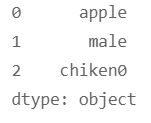import pandas

if  __name__ == '__main__':
data=pandas.Series()
print(type(data))
#print(data)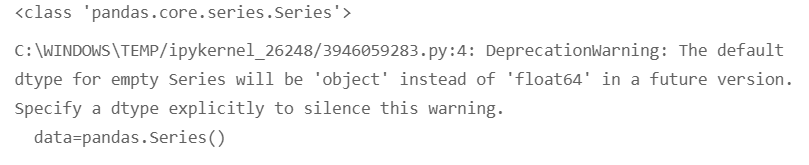import pandas

if  __name__ == '__main__':
data={'name':'小王','age':'18'}
print(pandas.Series(data))
print(pandas.Series(data)['name'])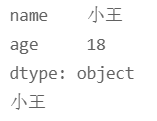三、DataFrame

二维数据结构，数据以行和列的表格的形式排列，可以结合xlwt模块处理excel表格 (想办法怎么把字典转换为列表格式)

用法:

pandas.DataFrame(data,index,columns,dtype,copy)

• data 数据采取各种形式
• index 对于行标签，要用于结果帧的索引是可选缺醒值 np.arange(n),如果没有索引被传递
• columns 对于列标签，可选的默认语法是 np.arange(n) 这只是在没有索引传递情况下才是这样
• dtype 每列的数据类型
• copy 复制数据，默认为false

写入 .xls 文件

写入 xls文件的两种方式

方法1：
write_xls(sheetname,data1,path) # data1 是列表

方法2：
df.pandas.DataFrame(data1) # data 是列表
df.to_excel(‘test.xls’)

import pandas
import xlwt

def write_xls(sheet_name,value,path):
index=len(value)
xls=xlwt.Workbook()                         #创建xls文件
for i in range(0,index):                    #写入数据
for j in range(0,len(value[i])):
table0.write(i,j,value[i][j])
xls.save(path)

if __name__ == '__main__':
data1=[['id','001'],['name','dog'],['age',5]]
#sheetname='test_sheet'
#path='test.xls'
df=pandas.DataFrame(data1)
df.to_excel('test.xls')
#write_xls(sheetname,data1,path)

DataFrame 数据的读取

从字典创建一个序列必须有 index=

import pandas

if __name__ == '__main__':
data={'id':'001','name':'dog','age':'3',
'id2':'002','name2':'cat','age2':'2'}       #创建一个字典
df=pandas.DataFrame(data,index=)       #将上面data数据，标记为 111行
print(df['name'])
print('-'*20)
print(df.iloc)       #打印第一行的数据
print('-'*20)
print(df.loc)      #打印出 行名是111 的那行数据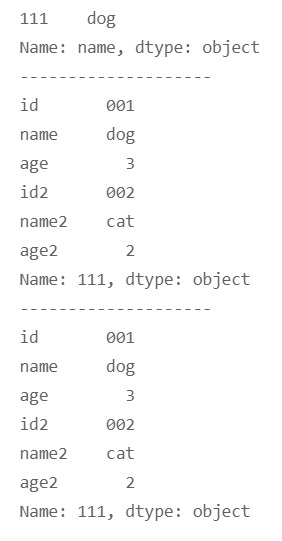参考

展开全文python 数据分析 pandas
• Python之Pandas模块讲解及案例 一、安装Pandas pip install Pandas 二、简介 Pandas是一个开源的，BSD许可的库，为Python (opens new window)编程语言提供高性能，易于使用的数据结构和数据分析工具。 Pandas是...

一、安装Pandas

pip install Pandas

二、简介

Pandas是一个开源的，BSD许可的库，为Python (opens new window)编程语言提供高性能，易于使用的数据结构和数据分析工具。

Pandas是NumFOCUS (opens new window)赞助的项目。这将有助于确保Pandas成为世界级开源项目的成功，并有可能捐赠 (opens new window)给该项目。

另外，获得 Pandas 的最佳方式是通过 conda(opens new window)：
conda install Pandas
但是此方法安装较慢，不太推荐，可以直接pip。

1、Series对象的实现

Series对象是相当于一个一维的数组
方法1

import pandas as pd
"""
导入pandas模块
"""
ser_0 = pd.Series(data=[0, 1, 2, 3, 4, 5])
# data 参数表示：所取得的数值是多少
print(ser_0)

# output>
0    0
1    1
2    2
3    3
4    4
5    5
dtype: int64
​# 第一列是索引（indexs）
# 第二列是数值（values）

方法2

import pandas as pd
ser_1 = pd.Series(data=[0, 1, 2, 3], index=['a', 'b', 'c', 'd'])
# index 表示自定义索引
print(ser_1)

# output>
a    0
b    1
c    2
d    3
dtype: int64

方法3

import pandas as pd
config = {
'one':1,
'two':2,
'three':3,
'four':4
}
# 字典
ser_2 = pd.Series(config)
print(ser_2)

# output>
one      1
two      2
three    3
four     4
dtype: int64

head() 与 tail() 用于快速预览 Series 与 DataFrame，默认显示 5 条数据，也可以指定显示数据的数量。
这里先以Series为例，DataFrame会在后续讲解。
下面将用到numpy模块，关于numpy模块的讲解可以参见我的另一篇博文：
python 之 numpy 模块详细讲解及其应用案例
链接：
https://blog.csdn.net/m0_54218263/article/details/114272263

import pandas as pd
import numpy as np
index_list = list('abcdefghijklmnopqrst')
data_list = np.arange(20)
ser = pd.Series(data=data_list, index=index_list)
print(ser.tail())
print(ser.tail(3))

# output>
a    0
b    1
c    2
d    3
e    4
dtype: int32

a    0
b    1
c    2
dtype: int32

p    15
q    16
r    17
s    18
t    19
dtype: int32

r    17
s    18
t    19
dtype: int32

3、属性与底层数据

index属性
values属性

import pandas as pd
ser = pd.Series(data=[0, 1, 2, 3, 4, 5, 6, 7, 8, 9, 10], index=['a', 'b', 'c', 'd', 'e', 'f', 'g', 'h', 'j', 'k', 'l'])
print(ser.index)
print(ser.values)

# output>
Index(['a', 'b', 'c', 'd', 'e', 'f', 'g', 'h', 'j', 'k', 'l'], dtype='object')
[ 0  1  2  3  4  5  6  7  8  9 10]

4、Series对象的切片操作

实际上，Series对象的切片与数组的切片差别并不大。

import pandas as pd
ser = pd.Series([1, 2, 3, 4, 5, 6, 7, 8, 9, 0, 11])
print(ser)
print(ser[1:7])
print(ser[0:8:2])
print(ser[:])
print(ser[-5:-2])

# output>
1

1    2
2    3
3    4
4    5
5    6
6    7
dtype: int64

0    1
2    3
4    5
6    7
dtype: int64

0      1
1      2
2      3
3      4
4      5
5      6
6      7
7      8
8      9
9      0
10    11
dtype: int64

6    7
7    8
8    9
dtype: int64

5、Series对象的一些常用方法

1、Series.index
索引
2、Series.values
数值
3、Series.drop(index)
删除某一个数据
4、Series.sum
求所有元素的和

1、DataFrame对象的实现

方法1

import pandas as pd
df = pd.DataFrame([[0, 1, 2, 3, 4], [5, 6, 7, 8, 9]])
print(df)方法2

import pandas as pd
df = pd.DataFrame(data=[[0, 1, 2, 3, 4], [5, 6, 7, 8, 9]], index=['a', 'b'])
print(df)方法3

import pandas as pd
config={
'a': [1, 2, 3, 4, 5],
'b': [4, 5, 6, 7, 8],
'c': [2, 4, 6, 8, 10]
}
df = pd.DataFrame(data=config,index=['one', 'two', 'three', 'four', 'five'])
print(df)2、DataFrame的取值以及切片

1、取值

import pandas as pd
config = {
'a': [0, 1, 2, 3, 4, 5, 6, 7],
'b': [8, 9, 10, 11, 12, 13, 14, 15],
'c': [3, 6, 9, 11, 13, 15, 17, 19],
'd': [2, 4, 6, 9, 12, 14, 16, 18,]
}
df = pd.DataFrame(data=config, index=['a', 'b', 'c', 'd', 'e', 'f', 'g', 'h'])
print(df)
print(df.tail())某一个位置处的值：

import pandas as pd
config = {
'a': [0, 1, 2, 3, 4, 5, 6, 7],
'b': [8, 9, 10, 11, 12, 13, 14, 15],
'c': [3, 6, 9, 11, 13, 15, 17, 19],
'd': [2, 4, 6, 9, 12, 14, 16, 18,]
}
df = pd.DataFrame(data=config, index=['a', 'b', 'c', 'd', 'e', 'f', 'g', 'h'])
print(df.a.a)
print(df.a.b)
print(df.c.f)
# 实际上是相当于取出字典中的数据的类似方法，
# 先去取一列，
# 再从这一列中去取出来某一行的数据点。

# output>
0
1
15

2、切片
1、行切片

import pandas as pd
config = {
'a': [0, 1, 2, 3, 4, 5, 6, 7],
'b': [8, 9, 10, 11, 12, 13, 14, 15],
'c': [3, 6, 9, 11, 13, 15, 17, 19],
'd': [2, 4, 6, 9, 12, 14, 16, 18,]
}
df = pd.DataFrame(data=config, index=['a', 'b', 'c', 'd', 'e', 'f', 'g', 'h'])
print(df[0:4])
# 从第零行切刀第三行数据
print(df['a':'c'])
# 从下标为 a 的行一直切到下标为 b 的行2、列切片

import pandas as pd
config = {
'a': [0, 1, 2, 3, 4, 5, 6, 7],
'b': [8, 9, 10, 11, 12, 13, 14, 15],
'c': [3, 6, 9, 11, 13, 15, 17, 19],
'd': [2, 4, 6, 9, 12, 14, 16, 18,]
}
df = pd.DataFrame(data=config, index=['a', 'b', 'c', 'd', 'e', 'f', 'g', 'h'])
print(df.a)
print(df.b)
print(df.c)

# output>

a    0
b    1
c    2
d    3
e    4
f    5
g    6
h    7
Name: a, dtype: int64

a     8
b     9
c    10
d    11
e    12
f    13
g    14
h    15
Name: b, dtype: int64

a     3
b     6
c     9
d    11
e    13
f    15
g    17
h    19
Name: c, dtype: int64

3、举一个例子，利用以上的类以及numpy来实现一个时间列表

import pandas as pd
import numpy as np
dates = pd.date_range('20130101', periods=6)
df = pd.DataFrame(np.random.randn(6, 4), index=dates, columns=list('ABCD'))
print(df)
print(df.dtypes)
# 查看类型
print(df.describe())
# 查看对数据的统计结果

# output>
A         B         C         D
2013-01-01 -1.177484  0.516432 -0.425316 -0.499715
2013-01-02 -0.128610 -1.052472 -0.151716 -0.019494
2013-01-03  0.257262 -0.857907  0.111126 -1.584577
2013-01-04  0.383851 -0.952546 -0.867269 -0.760178
2013-01-05  1.489054 -0.727922 -0.190620  0.230165
2013-01-06 -0.210085  1.699750 -0.100482 -0.784437

A    float64
B    float64
C    float64
D    float64
dtype: object

A         B         C         D
count  6.000000  6.000000  6.000000  6.000000
mean   0.102332 -0.229111 -0.270713 -0.569706
std    0.873513  1.106510  0.339157  0.641838
min   -1.177484 -1.052472 -0.867269 -1.584577
25%   -0.189716 -0.928886 -0.366642 -0.778372
50%    0.064326 -0.792915 -0.171168 -0.629947
75%    0.352204  0.205343 -0.113291 -0.139549
max    1.489054  1.699750  0.111126  0.230165这里就是一个简单的介绍了，也就是一个入门简介，更深入的学习可以参考官方文档，链接如下：
https://www.pypandas.cn/docs/getting_started/tutorials.html#%E5%AE%98%E6%96%B9%E6%8C%87%E5%8D%97
谢谢大家的阅读 ！！ ~~~

展开全文python pycharm 抽象代数 大数据 经验分享
• Pandas类似R语言中的数据框(DataFrame),Pandas基于Numpy,但是对于数据框结构的处理比Numpy要来的容易。1. Pandas的基本数据结构和使用Pandas有两个主要的数据结构：Series和DataFrame。Series类似Numpy中的一维数组...
• 这个非常简单，pandas内置了大量函数和类型，可以快速处理日常各种文件，下面我以txt，excel，csv，json和mysql这5种类型文件为例，简单介绍一下pandas是如何快速读取这些文件的：txt文件这是最常见的一种文本文件...
• pandas模块依赖于numpy模块(在pip安装pandas时会自动安装)，也需要引入import numpy as nppandas的数据结构有Series和DataFrameSeries是一维数据结构DataFrame表示为具有行和列的二维数据DataFrame的每个column...python
• pandas 2.1基础pandas import pandas as pd data_frame = pd.read_csv(input_file)#读取csv文件 data_frame.to_csv(output_file, index=False)#输出csv文件内容 2.2 筛选特定的行 2.2.1 行中的值满足某个条件 ...数据分析 python pandas
• 在对我的系统做了一些改动之后，...系统-Hyper-v 2016，Python 3.5.4，Pandas 0.23安装到c:\Python35。在问题-在不同的位置安装了以前的Python实例。在安装3.5.4之前，我删除了这些目录并删除了C:\Program Files...
• 结合金融时间序列演示Pandas模块的操作（三）  由于 Pandas 是 Python 的外部第三方模块，在使用前需要导入，并查询 Pandas 的版本号信息。 >>>import pandas as pd >>>pd.__version__ #查询...python 经验分享
• import pandas as pd # 导入pandas模块 s = pd.Series([1,2,3,4]) s > 0 1 1 2 2 3 3 4 dtype: int64 >>> s.index > RangeIndex(start=0, stop=4, step=1) 更改前面序号index >>>...python
• 基本概念 Series 是带有标签的一维数组，可以...import pandas as pd s = pd.Series(np.random.randint(5,size = 5)) print(s) print(type(s)) ================================ 0 1 1 1 2 4 3 1 4 4 dtype: int32pandas
• a, dtype: int32 <class 'pandas.core.series.Series'> a c one 35 17 two 53 51 three 82 51 <class 'pandas.core.frame.DataFrame'> # 按照index选择行，只选择一行输出Series，选择多行输出Dataframe data3 = df...python pandas
• 一、numpy模块NumPy(NumericPython)模块是Python的一种开源的数值计算扩展。这种工具可用来存储和处理大型矩阵，比Python自身的嵌套列表(nestedliststructure)结构要高效的多(该结构也可以用来表示矩阵(matrix))。...
• Pandas 数据结构 - Series Pandas Series 类似表格中的一个列（column），类似于一维数组，可以保存任何数据类型。 Series 由索引（index）和列组成，函数如下： pandas.Series( data, index, dtype, name, copy) ...python
• pandas模块

2021-12-10 11:39:37
Pandas简介 定义 一个开源的python类库，用于数据分析、数据处理、数据可视化特点 高性能、容易使用的数据结构、容易使用的数据分析工具pandas数据类型 Series是由相同数据类型组成的一维数组。 DataFrame：二维的...python
• 结合金融时间序列演示Pandas模块的操作（一）  在金融分析与风险管理中，往往需要对金融时间序列进行分析。时间序列就是以时间作为索引的数据集合，但 NumPy 的数组结构无法满足时间序列的要求。因此，需要引入 ...
• 文章目录一、前言二、pandas是什么？三、使用步骤1.引入库2.读入数据四、代码 一、前言 继上次的更新有三个月了，这次带来了最新的mysql查询并写入excel表格不同sheet页的方法，也算是自我的一个提高总结，同时也...mysql sql pandaspython
• sci = s[['a','b','e']] print(sci,type(sci)) ======================================= a 0.511722 b 0.314775 e 0.965524 dtype: float64 <class 'pandas.core.series.Series'> 切片索引 如果用下标去做切片，...pandas
• 这有点类似于内置函数 sorted() 函数里面的 key 函数 Help on method sort_index in module pandas.core.frame: sort_index(axis=0, level=None, ascending: 'Union[Union[bool, int], Sequence[Union[bool, int]]]'...python pandas 数据分析
• pandas模块、mplfinance模块和matplotlib模块介绍pandas模块pandas为解决数据分析任务而创建，纳入了大量的库和一些标准的数据模型，简而言之，它提供了很多数据处理的方法，此文就是借用它来生成DataFrame格式的...
• 一、pandas模块 pandas（Python Data Analysis Library）是基于numpy的数据分析模块，提供了大量标准数据模型和高效操作大型数据集所需要的工具，可以说pandas是使得Python能够成为高效且强大的数据分析环境的重要...数据挖掘 数据分析 python
• 基于pandas的一些金融常用基本操作 一、数据结构 1. 序列 Series 序列一般只有两列，一列是索引 index，一列是数据。 # 手动输入方式创建 return_series1 = pd.Series([0.003731, -0.001838, -0.003087, -0.024112],...python
• 健壮的数据处理模块Pandas，能够处理数据的预处理作业，如数据类型的转化、缺失值的处理、描绘性核算分析和数据的汇总等一、序列与数据框的结构Pandas模块的中心操作政策为序列和数据框。序列指数据会集的一个字段，......